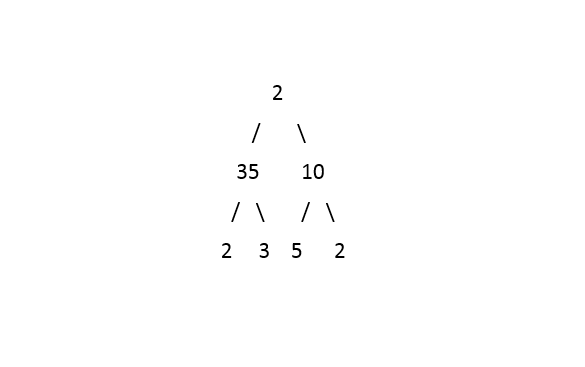1

# Leaf And Path

Difficulty: MEDIUMContributed By
Ayush Thakur
Avg. time to solve
35 min
Success Rate
65%

Problem Statement
Suggest Edit

#### For example:

``````If the path is 1 -> 20 -> 8 -> 721, then the sequence obtained is “1208721”.
``````

#### Leaf traversal is the sequence of leaves of the tree traversed from left to right.

##### Input Format :
``````The first line of input contains an integer 'T' representing the number of test cases. Then the test cases follow :

The only line of each test case contains elements in the level order form. The line consists of values of nodes separated by a single space. In case a node is null, we take -1 on its place.

For example, the input for the tree depicted in the below image would be :
````````````1
2 3
4 -1 5 6
-1 7 -1 -1 -1 -1
-1 -1
``````

#### Explanation :

``````Level 1 :
The root node of the tree is 1

Level 2 :
Left child of 1 = 2
Right child of 1 = 3

Level 3 :
Left child of 2 = 4
Right child of 2 = null (-1)
Left child of 3 = 5
Right child of 3 = 6

Level 4 :
Left child of 4 = null (-1)
Right child of 4 = 7
Left child of 5 = null (-1)
Right child of 5 = null (-1)
Left child of 6 = null (-1)
Right child of 6 = null (-1)

Level 5 :
Left child of 7 = null (-1)
Right child of 7 = null (-1)

The first not-null node(of the previous level) is treated as the parent of the first two nodes of the current level. The second not-null node (of the previous level) is treated as the parent node for the next two nodes of the current level and so on.
The input ends when all nodes at the last level are null(-1).
``````
##### Note :
``````The above format was just to provide clarity on how the input is formed for a given tree.
The sequence will be put together in a single line separated by a single space. Hence, for the above-depicted tree, the input will be given as:

1 2 3 4 -1 5 6 -1 7 -1 -1 -1 -1 -1 -1
``````
##### Output Format :
``````For each test case, print “True” if a path with the same representation as the leaf traversal exists, else “False”.

The output of each test case will be printed in a separate line.
``````
##### Note :
``````You don't need to print anything. It has already been taken care of, just implement the given function.
``````
##### Constraints :
``````1 <= T <= 2*10^2
0 <= N <= 3*10^3
0 <= NODE.DATA <= 10^5

Time Limit: 1 sec
``````
##### Sample Input 1 :
``````1
2 35 10 2 3 5 2 -1 -1 -1 -1 -1 -1 -1 -1 -1 -1
``````
##### Sample Output 1 :
``````True
``````
##### Explanation For Sample Input 1 :``````The leaf traversal for the above binary tree is “2352”. The path 2->35->2 has the same representation as the leaf traversal i.e. “2352”. Thus, the answer is true.
``````
##### Sample Input 2 :
``````2
1 2 3 4 5 -1 7 -1 -1 -1 -1 -1 -1
5 -1 -1
``````
##### Sample Output 2 :
``````False
True
``````Console